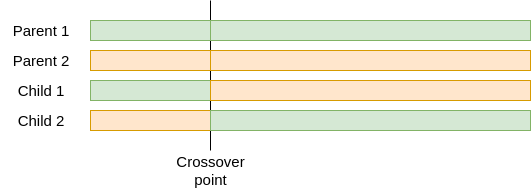# What is a genetic algorithm (and how does it work)?

Sep 16, 2021 by Alexandre Croix | 1756 views

In the Machine Learning field, there are plenty of different algorithms. Each algorithm has its own advantages and drawbacks, its domains of application and its performance. One of these algorithms we heard the most about is the Genetic Algorithm.

Genetic Algorithm... It is a fancy term, a buzzword. Perhaps this is one reason why we hear (or at least we remember) a lot about this term. But what exactly is a Genetic Algorithm?

## Definition

A Genetic Algorithm is a Machine Learning algorithm. That means its purpose is to learn and improve from experience how to do a specific task in an autonomous way (without being explicitly programmed). These kinds of algorithms imitate the way humans learn, gradually improving their accuracy to perform a task.

A Genetic Algorithm is an evolutive process that tries to find a solution to minimize (or maximize) a given function.In the previous figure, if the algorithm tries to minimize the function, the Genetic Algorithm will try to find the global minimum point. Of course, this example is really easy because the function has only one parameter.

Practically, the algorithm tries to find a global minimum (or maximum) for any n parameters functions: where: are function parameters.

It is called Genetic because its principle is inspired by biological gene reproduction.

## Genetic Algorithm structure

A Genetic Algorithm is an evolutive process that maintains a population of chromosomes (potential solutions). Each chromosome is composed of several characteristics called genes. The all process has 5 main steps:

• Initial population generation:
• Fitness score evaluation
• Selection
• Crossover
• MutationThe fitness score evaluation, selection, crossover and mutation will be repeated until a stop condition. This condition can be a specific number of iterations (called generation) or a threshold reached (satisfying result).

### Initial population generation

The first step is the initial population generation. A population contains a number of chromosomes. A chromosome is a potential solution and is composed of several characteristics (called genes). Each function parameter is a gene. So, for a function with 6 parameters, a chromosome has 6 genes (one for each parameter). The following figure is a representation of a 4 element population. Each chromosome contains 6 genes.Usually, the initial population has a fixed size and is generated randomly. The population size is one important parameter for Genetic Algorithms.

### Fitness score evaluation

Each chromosome from the population is evaluated to obtain a fitness score. Better is the solution, better will be its fitness score. The probability that a chromosome will be selected for reproduction is based on its fitness score. Concretely, the chromosome genes are used as parameters for the function the algorithm tries to minimize (or maximize). It is the crucial part of the algorithm: how to evaluate correctly the quality of a solution (set of parameters)? Without a good fitness score evaluation function, the Genetic Algorithm will not produce good results.

### Selection

Based on the fitness score, a percentage of the population will be selected for reproduction. The better is the fitness score, the higher is the probability to be selected. A second important parameter for Genetic Algorithms is the crossover rate. The crossover rate represents the percentage of the population selected from generation t to generation t+1 for reproduction.

### Crossover

The crossover step is the most related to biological reproduction. The chromosomes selected from the previous generation are mixed, two-by-two, to generate new chromosomes called children. These new chromosomes contain some elements from their two parents.New chromosomes are generated until the population is complete (population number reaches). The bigger is the crossover rate, the fewer new chromosomes are generated.

### Mutation

The mutation step mimics the mutation during genetic reproduction. Each chromosome has a probability to mutate. If a mutation occurs, a random gene in the chromosome will be changed to another random value.The mutation rate is another important parameter: it represents the probability that a chromosome mutates.

This step is very important to obtain good results. Indeed, a Genetic Algorithm can be stuck in a local minimum (maximum). The mutation is a "jump" to another point in the solution space.The figure illustrates what happened during a mutation for a simple 1 parameter function.

## Genetic Algorithm Hyper-parameters

As described earlier, a Genetic Algorithm has several parameters that can be tuned to obtain better results. The set of parameters is called Hyper-parameters. The most important hyper-parameters are:

• Population size: number of chromosomes in the population. This parameter impacts the algorithm running time. The more chromosomes in the population the longer the algorithm will take.
• Crossover rate: percentage of chromosomes selected from generation t to be used in generation t+1 for reproduction.
• Mutation rate: probability that a chromosome mutates.
• Generation number: number of iterations of the algorithm before it stops. The running time is also directly impacted by this parameter.

## Conclusion

This article describes the basics of a Genetic Algorithm. In practice, the algorithms can be more complex, have more parameters,... For example, there exist several methods for the Selection step (Roulette Wheel Selection, Tournament Selection,...) or for the Crossover step.

It is not possible to be sure the algorithm returns the best solution possible. The algorithm tries to find a minimum (maximum) but it is possible it does not explore an area from the solution space. It is very important to test different Hyper-parameters sets. The parameters depend on the data and on the problem you try to solve.

This Genetic Algorithm can work well and produce good results even with a medium-sized dataset. This is not the case for a Deep-learning algorithm which requires a lot of data.